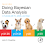## Sunday, June 16, 2013

### Bayesian robust regression for Anscombe quartet

In 1973, Anscombe presented four data sets that have become a classic illustration for the importance of graphing the data, not merely relying on summary statistics. The four data sets are now known as "Anscombe's quartet." Here I present a Bayesian approach to modeling the data. The Anscombe quartet is used here as merely another illustration of two frequently made points in Bayesian analysis: JAGS/BUGS is a very flexible language for expressing a variety of models (e.g., robust non-linear regression), and it is important to conduct a posterior predictive check of the descriptive adequacy of the model.

(I first messed around with analyzing Anscombe's quartet back in October 2010, for personal interest. A conversation I had last week in Basel, Switzerland, suggested that this analysis might interest a larger audience. Hence this blog post.)

Below are the four data sets, shown as black circles. They are modeled with typical linear regression that assumes normality:
y ~ dnorm( b0+b1*x , sigma )
with vague priors on the intercept b0, the slope b1, and the standard deviation sigma.

Actually, for easy consistency with the subsequent analysis, I used a t distribution with the normality (df) parameter given a prior that insists it is very large, near 500, which is essentially normal. Thus, the model is
y ~ dt( b0+b1*x , sigma , nu )
with vague priors on b0, b1, and sigma, and the prior on normality being
nu ~ dnorm( 500 , sd=0.1 )

The figures below show the data in the left panels, with a smattering of credible regression lines superimposed, and veritical bars that indicate the 95% HDI of posterior predicted y values at selected x values:

Notice that the marginal parameter estimates are the same for all four data sets (up to MCMC sampling error), which is exactly the point that Anscombe wanted to emphasize for least-squares regression. Here we see that it is also true for Bayesian estimation, and this is no surprise when we remember from formal Bayesian analysis of linear regression (with normally distributed noise) that the "sufficient statistics" of the  data determine the posterior distribution, and the sufficient statistics are identical for the four data  sets.

One of the beauties of the flexibility of JAGS/BUGS is that it is easy to implement other models. In particular, it is easy to implement regression that is robust to outliers, using the t distribution. I used the same model as above,

y ~ dt( b0+b1*x , sigma , nu )
with vague priors on b0, b1, and sigma, but I put a vague prior on the normality of the t distribution:
nu <- nuMinusOne + 1
nuMinusOne ~ dexp( 1/29 )
This is the same prior on nu as is used in the "BEST" model for robust comparison of two groups. The resulting posteriors look like this:

The normality parameter (nu) is not much different from its prior for Types 1, 2, and 4, resulting in slightly smaller estimates of sigma than when forcing nu to be 500.  Most notably, however, for Type 3, the model detects that the data fall on a line and have a single outlier, indicated by the SD (sigma) estimated as an extremely small value, and the normality (nu) hugging 1.0, which is its smallest possible.

Analogously, we could easily do a robust quadratic trend analysis, and thereby detect the non-linear trend in Type 2. See this previous blog post for how to extend a JAGS/BUGS linear regression to include a quadratic trend.

To reiterate and conclude, the Anscombe quartet not only makes its original point, that we should look at the posterior predictions to make sure that the model is a reasonable description of the data, but it also highlights that for Bayesian analysis using JAGS/BUGS, it is easy to try other models that may be better descriptions of the data.
ADDED 18 JUNE 2013: Rasmus Baath, after reading the above post, has actually implemented the extensions that I merely suggested above. Please continue reading his very interesting follow up.

1.This comment has been removed by the author.

2.Nice post! For fun I tried to extend your model to, in some sense, model all four datasets in Anscombe's quartet. Perhaps not useful, but it was a fun exercise! Wrote a post about it here:

http://www.sumsar.net/2013/06/bayesian-modeling-of-anscombes-quartet/

3.Very interesting! Thanks! (I edited the post to highlight your follow up.)

4.Sorry to bother, I just rolled out a new version of my home page and the link to the "continuation" of you post has unfortunately automatically changed to:

http://www.sumsar.net/blog/2013/06/bayesian-modeling-of-anscombes-quartet/

/Rasmus